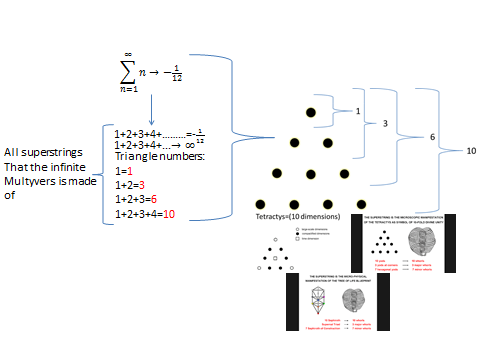top of page
Search

# Tree of life 64 tetrahedron grid part 1

(8 tree of life's)=(64 tetrahedron grid)=(2 tetragrammatons)(Tetragrammaton)=(4 tree of life's)=36+1

36+1=36:1=36:36=49:49=77:77=28444=161616=777=7:77=7:2

7:2=tetragrammaton

444=4³=(64 tetrahedron grid)

Tree of life superstring: